In :
import numpy as np
import matplotlib.pyplot as plt
from IPython.display import Image
import pandas as pd
from matplotlib import cm


### Lecture 25:¶

• Learn about 3D plots of points and surfaces
• Show some examples with subduction zone Earthquakes and isotopic systems

### 3D Plotting with Python¶

Contour plots are really just a way to visualize something that is inherently 3D on a 2D surface. Think about our topographic map - the contour intervals are elevations and our brains can reconstruct the 3D world by looking at the contours on the map. But with computers we can visualize the 3D world in a more realistic manner. There are many 3D plotting packages that apply many different approaches to plot in 3D. For example, mplot3d, is a 3D toolkit of matplotlib that uses the same logic as for "regular" matplotlib. For more on this module, see:

http://matplotlib.sourceforge.net/mpl_toolkits/mplot3d/index.html

But for more 3D horsepower, there is a module called mlab, which is part of the mayavi package. See:

http://github.enthought.com/mayavi/mayavi/mlab.html

And then there is Mayavi itself, which comes with the Canopy Python Edition. This was way beyond what I know, but if you are curious, check out this website:

http://github.enthought.com/mayavi/mayavi/examples.html

### 3D Plotting in Jupyter Notebooks¶

Until now, we have used %matplotlib inline to plot our matplotlib figures within the jupyter notebook. In order to plot a 3D figure within the jupyter notebook and allow interaction with a 3D plot, we must use this alternative: %matplotlib notebook

## Plotting points in 3D¶

Remember this plot from Lecture 18?

In :
Image(filename='Figures/earthquakes_depth.png')

Out: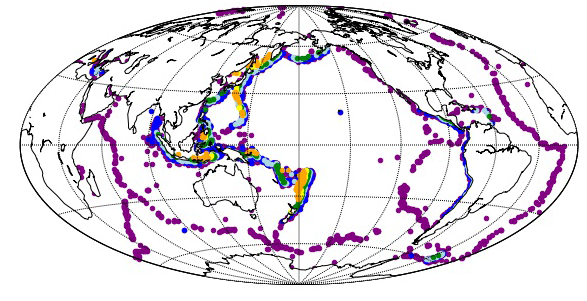We used color to represent the depth of each earthquake . You can see that there are increasingly deep earthquakes as you move away from subduction zones- sort of.

It would be more instructive to view the depth of the earthquakes as points in a 3D plot. So, let's read-in the data, filter for a lat/lon box from 35$^{\circ}$S to 20$^{\circ}$N and 170$^{\circ}$-190$^{\circ}$E (the Marianas trench), and plot the data in 3D.

To begin, let's filter the data by the desired bounds and make an array of longitudes,x, an array of latitudes,y,and an array of depths,z.

In :
# read in the data from the Lecture 18 as a Pandas DataFrame:

# define some boundaries for our box
lat_min,lon_min,lat_max,lon_max=-35,175,-15,190

# use Pandas filtering to fish out lat/lon in this range
box=eq_data[(eq_data.longitude.values%360<lon_max)&(eq_data.longitude.values%360>=lon_min)]
box=box[(box.latitude.values<lat_max)&(box.latitude.values>=lat_min)]

# and export them to NumPy arrays
x=box.longitude.values%360
y=box.latitude.values
z=-box.depth.values


We can use the Axes3D class from the mplot3d, which is another tool in the matplotlib toolkit (mpl_toolkits). [Remember that is where we got Basemap.]

We will import the Axes3D module.

But before we can use it, we have to make the notebook ready for 3D plots. One somewhat annoying thing about using the 3D options in a Jupyter notebook is that there are two different magic commands that are used. You are already familiar with the %matplotlib inline command that allows us to plot "regular" matplotlib plots in the notebook. But now we need a NEW one: %matplotlib notebook strictly for plotting 3D objects. The trouble comes when you try to do both in one notebook, because you have to always use the magic correct command before you can see your figure. So we import the Axes3D module and call the magic command:

%matplotlib notebook

In :
# import the module
from mpl_toolkits.mplot3d import Axes3D
%matplotlib notebook


The trick to using Axes3D is to first make a figure object using plt.figure( ) (called fig below) and then use the figure method fig.add_subplot( ) to make an Axis instance (here called ax). Finally we set the keyword projection of the add_sublot call to '3d'.

In :
fig=plt.figure(1,(7,7)) # we need to make a figure object
ax = fig.add_subplot(111, projection='3d'); # so we can do this.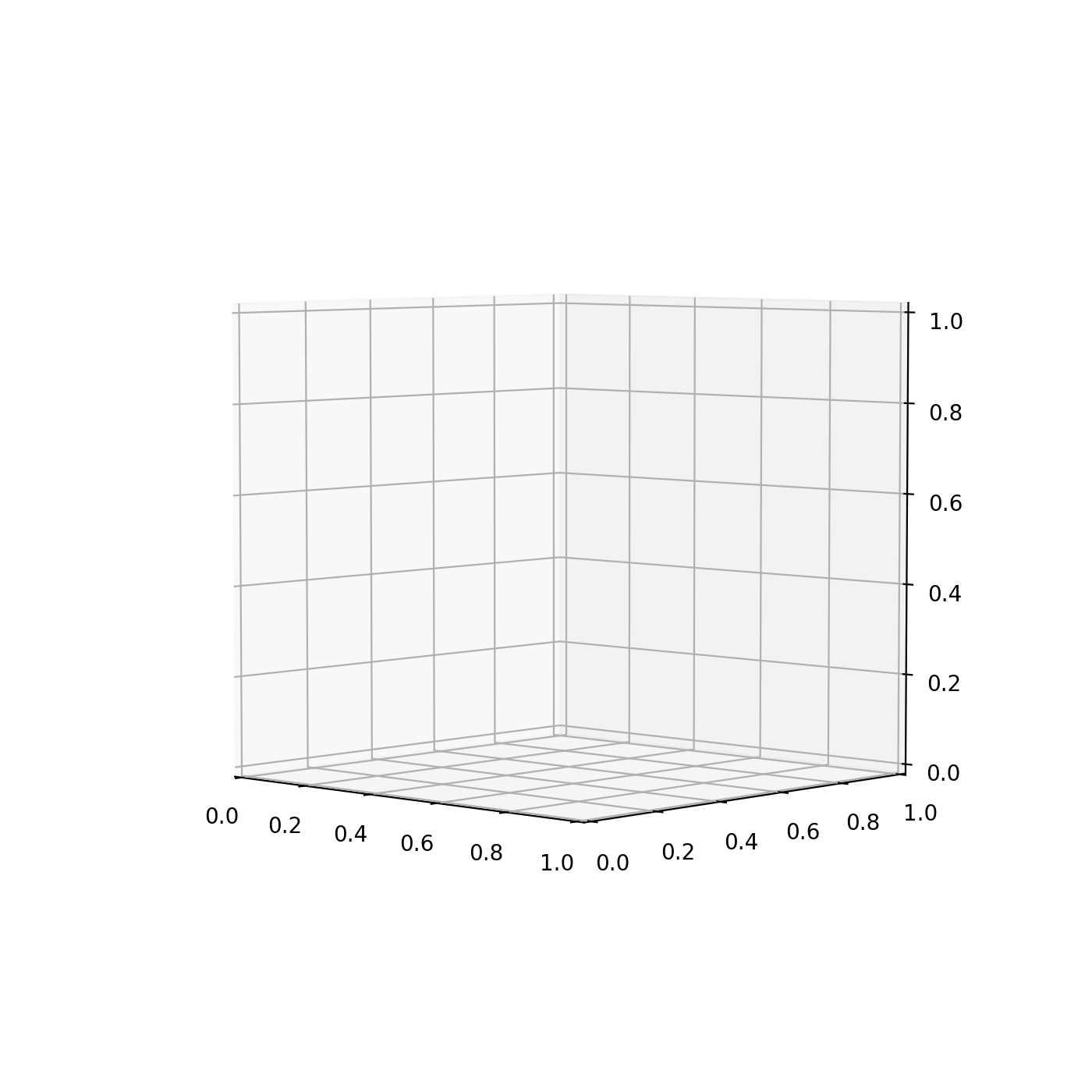This makes an empty set of axes. But you can twirl them around! [Okay, I thought that was cool.]

Now we can decorate the plot with some data.

In :
fig=plt.figure(2,(7,7)) # let's make a new one.
ax = fig.add_subplot(111, projection='3d') # so we can do this.

ax.scatter(x,y,z,c='r',marker='o')
ax.set_xlabel('Longitude')
ax.set_ylabel('Latitude')
ax.set_zlabel('Depth (km)');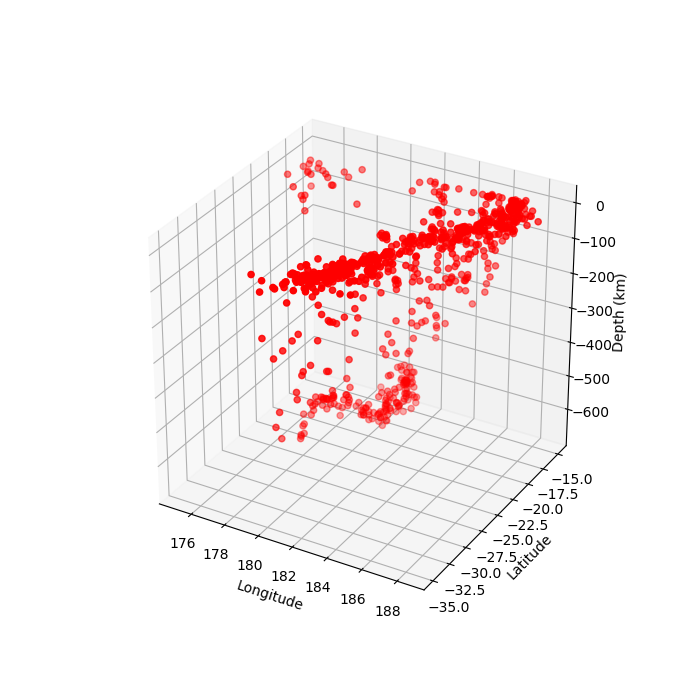Try twirling the figure around! Can you see the slab?

You can imagine other enhancements, for example setting the size of the points to be proportional to the size of the earthquake.

### 3D contour type plots¶

In Lecture 19, we learned how to make 2D color contour plots, but 3D is much more fun, so let's try to make a 3D version of something geophysical, namely the gravity anomaly of a buried sphere.

Let's choose a sphere with a radius $R$ of 2 m (whose volume is ${{4\pi}\over{3}}R^3$), that is buried $z=$ 3 m deep. The sphere's density ($\Delta \rho$) is 500 kg/m$^3$, which is, for this purpose, much higher than the surrounding material. Oh and we'll need the universal gravitational constant $G$, which is 6.67x 10$^{-11}$ Nm$^2$/kg$^2$.

The formula for gravitational attraction of such a body is:

$$g= {{4\pi}\over{3}} R^3 {{G \Delta \rho}\over{(h^2+z^2})},$$

where $h$ is the horizontal distance from the mass.

The units (from dimensional analysis remembering that Newtons are kg $\cdot$ m $\cdot$ s$^2$) are m $\cdot$ s$^{-2}$. 1 Gal (for Galileo) = 1 cm $\cdot$s$^{-2}$, so to convert $g$ to the units of microgals, we multiply the above by 10$^{8}$.

We can write our equation as a lambda function.

In :
gravity= lambda G,R,drho,h,z : (1e8*G*4.*np.pi*R**3.*drho)/(3.*(h**2+z**2)) # gravitational attraction.


And set up the arrays the same way as we did in the previous lectures as a color contour on a 2D surface.

In :
x=np.arange(-6.,6.,0.1) # range of x values
y=np.arange(-6,6.,0.1) # range of y values
X,Y=np.meshgrid(x,y) # make a meshgrid


To get the gravity array $g$, we need $z,G,R$ and $\Delta \rho$ and $h$ the horizontal distance from ground zero of the buried sphere, which is given by good old Pythagorous as:

$$h=\sqrt {x^2 + y^2}.$$

In :
# define the other parameters
z=3.
G=6.67e-11 # grav constant in Nm^2/kg^2 (SI)
drho=500 # density contrast in kg/m^3

h=np.sqrt(X**2+Y**2) # get the horizontal distance from ground zero for x,y
# and make the g array
g=gravity(G,R,drho,h,z) # multipy by a million to get the units reasonable for the plots.


We want to make the plot of the gravitational attraction first using our old friend from the Lecture 19, plt.pcolormesh( ).

In :
# put this back to 'normal' for use in the notebook using the correct Jupyter magic command
%matplotlib inline
plt.figure(3)
plt.axis('equal')
plt.axis('off');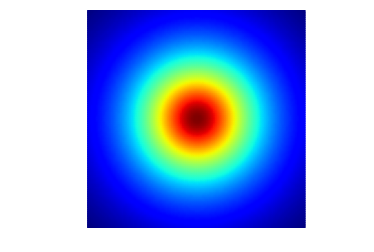Well that IS pretty. But now we want to do this in 3D.

### 3D Surfaces¶

There are a few ways to plot this in 3D: wireframes and surfaces. Let's start with a wireframe plot. For this we need to use matplotlib Axes3D.

#### Wireframes¶

There is an Axes3D method called plot_wireframe( ) which will do the trick. It is in many ways similar to the pcolormesh( ), but instead of using color to represent values, it uses a 3D projection.
Let's try the plot_wireframe( ) function on our gravity data.

In the following plot, we create an Axes3D instance called ax from the figure object, fig. By setting $projection='3d'$, we get a 3D projection.

Remember that because we were just plotting in 2D (%matplotlib inline), we have to call the 3D version (%matplotlib notebook).

In :
# makes a 3D plot in a notebook
%matplotlib notebook
fig=plt.figure(4,(8,5)) # make a figure object
ax=fig.add_subplot(111,projection='3d') # give it the powers of an Axes3D object
surf=ax.plot_wireframe(X,Y,g) # use plot_wireframe to do the plot.
ax.set_xlabel('X') # and label the axes
ax.set_ylabel('Y')
ax.set_zlabel('Z');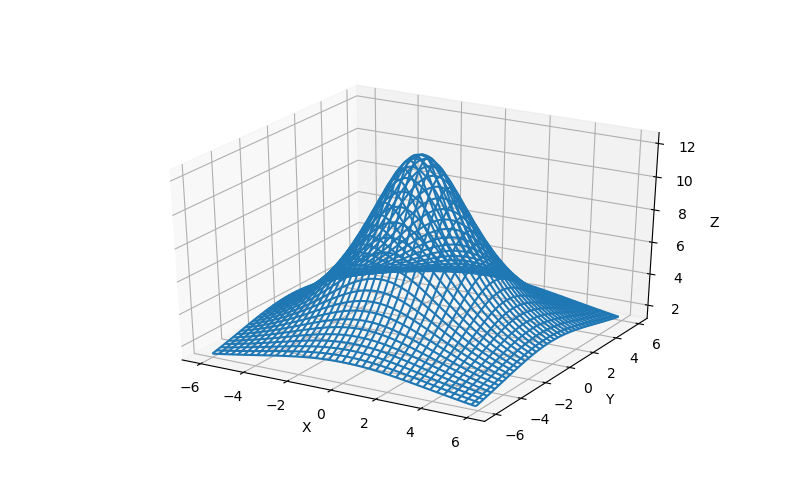Try twirling it around - pretty cool, huh?

#### surfaces¶

There is a related method plot_surface( ) which is also nice. We then call plot_surface( ) on the Axes3D instance and set labels to the 3 axis. Note that if we assign the surface instance to a variable, e.g., surf, we can do other things to it, like add a color bar. Finally, please admire the use of a colormap (cmap=cm.rainbow).

This works pretty much like plot_wireframe( ) but this one we can put a color bar on too.

In :
 # make the notebook ready for interactive 3D plots again
%matplotlib notebook
fig=plt.figure(4,(8,5)) # make a figure object
ax=fig.add_subplot(111,projection='3d') # get a 3d plot object
surf=ax.plot_surface(X,Y,g,cmap=cm.jet) # use plot_surface to do the plot using a color map
ax.set_xlabel('X') # and label the axes
ax.set_ylabel('Y')
ax.set_zlabel('Z')
bar=fig.colorbar(surf) # put on the color bar
bar.set_label('Gravity in $\mu$gal'); #label the color bar